University Physics Volume 1

# Chapter 4

4.1

(a) Taking the derivative with respect to time of the position function, we have $v→(t)=9.0t2i^andv→(3.0s)=81.0i^m/s.v→(t)=9.0t2i^andv→(3.0s)=81.0i^m/s.$ (b) Since the velocity function is nonlinear, we suspect the average velocity is not equal to the instantaneous velocity. We check this and find
$v→avg=r→(t2)−r→(t1)t2−t1=r→(4.0s)−r→(2.0s)4.0s−2.0s=(194i^−24i^)m2.0s=84i^m/s,v→avg=r→(t2)−r→(t1)t2−t1=r→(4.0s)−r→(2.0s)4.0s−2.0s=(194i^−24i^)m2.0s=84i^m/s,$
which is different from $v→(3.0s)=81.0i^m/s.v→(3.0s)=81.0i^m/s.$

4.2

The acceleration vector is constant and doesn’t change with time. If a, b, and c are not zero, then the velocity function must be linear in time. We have $v→(t)=∫a→dt=∫(ai^+bj^+ck^)dt=(ai^+bj^+ck^)tm/s,v→(t)=∫a→dt=∫(ai^+bj^+ck^)dt=(ai^+bj^+ck^)tm/s,$ since taking the derivative of the velocity function produces $a→(t).a→(t).$ If any of the components of the acceleration are zero, then that component of the velocity would be a constant.

4.3

(a) Choose the top of the cliff where the rock is thrown from the origin of the coordinate system. Although it is arbitrary, we typically choose time t = 0 to correspond to the origin. (b) The equation that describes the horizontal motion is $x=x0+vxt.x=x0+vxt.$ With $x0=0,x0=0,$ this equation becomes $x=vxt.x=vxt.$ (c) Equation 4.16 through Equation 4.18 and Equation 4.19 describe the vertical motion, but since $y0=0andv0y=0,y0=0andv0y=0,$ these equations simplify greatly to become $y=12(v0y+vy)t=12vyt,y=12(v0y+vy)t=12vyt,$$vy=−gt,vy=−gt,$$y=−12gt2,y=−12gt2,$ and $vy2=−2gy.vy2=−2gy.$ (d) We use the kinematic equations to find the x and y components of the velocity at the point of impact. Using $vy2=−2gyvy2=−2gy$ and noting the point of impact is −100.0 m, we find the y component of the velocity at impact is $vy=44.3m/s.vy=44.3m/s.$ We are given the x component, $vx=15.0m/s,vx=15.0m/s,$ so we can calculate the total velocity at impact: v = 46.8 m/s and $θ=71.3°θ=71.3°$ below the horizontal.

4.4

The golf shot at $30°.30°.$

4.5

134.0 cm/s

4.6

Labeling subscripts for the vector equation, we have B = boat, R = river, and E = Earth. The vector equation becomes $v→BE=v→BR+v→RE.v→BE=v→BR+v→RE.$ We have right triangle geometry shown in Figure 04_05_BoatRiv_img. Solving for $v→BEv→BE$, we have
$vBE=vBR2+vRE2=4.52+3.02vBE=vBR2+vRE2=4.52+3.02$
$vBE=5.4m/s, θ=tan−1(3.04.5)=33.7°.vBE=5.4m/s, θ=tan−1(3.04.5)=33.7°.$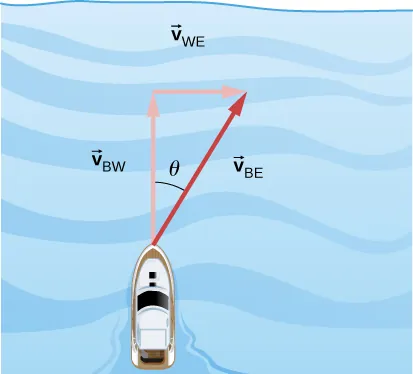### Conceptual Questions

1.

straight line

3.

The slope must be zero because the velocity vector is tangent to the graph of the position function.

5.

No, motions in perpendicular directions are independent.

7.

a. no; b. minimum at apex of trajectory and maximum at launch and impact; c. no, velocity is a vector; d. yes, where it lands

9.

They both hit the ground at the same time.

11.

yes

13.

If he is going to pass the ball to another player, he needs to keep his eyes on the reference frame in which the other players on the team are located.

15.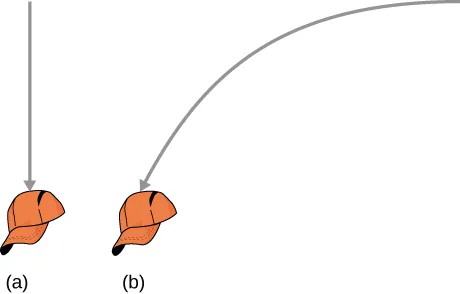### Problems

17.

$r → = 1.0 i ^ − 4.0 j ^ + 6.0 k ^ r → = 1.0 i ^ − 4.0 j ^ + 6.0 k ^$

19.

$Δ r → Total = 472.0 m i ^ + 80.3 m j ^ Δ r → Total = 472.0 m i ^ + 80.3 m j ^$

21.

$Sum of displacements = −6.4 km i ^ + 9.4 km j ^ Sum of displacements = −6.4 km i ^ + 9.4 km j ^$

23.

a. $v→(t)=8.0ti^+6.0t2k^,v→(0)=0,v→(1.0)=8.0i^+6.0k^m/sv→(t)=8.0ti^+6.0t2k^,v→(0)=0,v→(1.0)=8.0i^+6.0k^m/s$,
b. $v→avg=4.0​i^+3.0k^m/sv→avg=4.0​i^+3.0k^m/s$

25.

$Δr→1=20.00mj^,Δr→2=(2.000×104m)(cos30°i^+sin30°j^)Δr→1=20.00mj^,Δr→2=(2.000×104m)(cos30°i^+sin30°j^)$
$Δr→=1.700×104mi^+1.002×104mj^Δr→=1.700×104mi^+1.002×104mj^$

27.

a. $v→(t)=(4.0ti^+3.0tj^)m/s,v→(t)=(4.0ti^+3.0tj^)m/s,$$r→(t)=(2.0t2i^+32t2j^)mr→(t)=(2.0t2i^+32t2j^)m$,
b. $x(t)=2.0t2m,y(t)=32t2m,t2=x2⇒y=34xx(t)=2.0t2m,y(t)=32t2m,t2=x2⇒y=34x$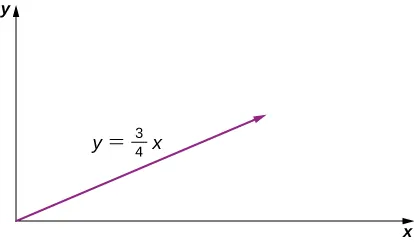29.

a. $v→(t)=(6.0ti^−21.0t2j^+10.0t−3k^)m/sv→(t)=(6.0ti^−21.0t2j^+10.0t−3k^)m/s$,
b. $a→(t)=(6.0i^−42.0tj^−30t−4k^)m/s2a→(t)=(6.0i^−42.0tj^−30t−4k^)m/s2$,
c. $v→(2.0s)=(12.0i^−84.0j^+1.25k^)m/sv→(2.0s)=(12.0i^−84.0j^+1.25k^)m/s$,
d. $v→(1.0s)=6.0i^−21.0j^+10.0k^m/s,|v→(1.0s)|=24.0m/sv→(1.0s)=6.0i^−21.0j^+10.0k^m/s,|v→(1.0s)|=24.0m/s$
$v→(3.0s)=18.0i^−189.0j^+0.37k^m/s,v→(3.0s)=18.0i^−189.0j^+0.37k^m/s,$$|v→(3.0s)|=190m/s|v→(3.0s)|=190m/s$,
e. $r→(t)=(3.0t2i^−7.0t3j^−5.0t−2k^)mr→(t)=(3.0t2i^−7.0t3j^−5.0t−2k^)m$
$v→avg=9.0i^−49.0j^+3.75k^m/sv→avg=9.0i^−49.0j^+3.75k^m/s$

31.

a. $v→(t)=−sin(1.0t)i^+cos(1.0t)j^+k^v→(t)=−sin(1.0t)i^+cos(1.0t)j^+k^$, b. $a→(t)=−cos(1.0t)i^−sin(1.0t)j^a→(t)=−cos(1.0t)i^−sin(1.0t)j^$

33.

a. $t=0.55st=0.55s$, b. $x=110mx=110m$

35.

a. $t=0.24s,d=0.28mt=0.24s,d=0.28m$, b. They aim high.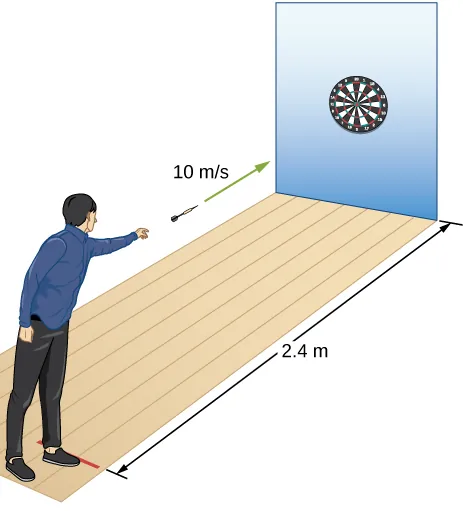37.

a., $t=12.8s,x=5619mt=12.8s,x=5619m$ b. $vy=125.0m/s,vx=439.0m/s,|v→|=456.0m/svy=125.0m/s,vx=439.0m/s,|v→|=456.0m/s$

39.

a. $vy=v0y−gt,t=10s,vy=0,v0y=98.0m/s,v0=196.0m/svy=v0y−gt,t=10s,vy=0,v0y=98.0m/s,v0=196.0m/s$,
b. $h=490.0m,h=490.0m,$
c. $v0x=169.7m/s,x=3394.0m,v0x=169.7m/s,x=3394.0m,$
d. $x=169.7m/s(15.0s)=2550m y=(98.0m/s)(15.0s)–4.9(15.0s)2=368m sr=2550mi^+368mj^x=169.7m/s(15.0s)=2550m y=(98.0m/s)(15.0s)–4.9(15.0s)2=368m sr=2550mi^+368mj^$

41.

$−100m=(−2.0m/s)t−(4.9m/s2)t2,−100m=(−2.0m/s)t−(4.9m/s2)t2,$ $t=4.3s,t=4.3s,$$x=86.0mx=86.0m$

43.

$R M o o n = 48 m R M o o n = 48 m$

45.

a. $v0y=24m/sv0y=24m/s$$vy2=v0y2−2gy⇒h=29.3mvy2=v0y2−2gy⇒h=29.3m$,
b. $t=2.4sv0x=18m/sx=43.2mt=2.4sv0x=18m/sx=43.2m$,
c. $y=−100my0=0y=−100my0=0$ $y−y0=v0yt−12gt2−100=24t−4.9t2y−y0=v0yt−12gt2−100=24t−4.9t2$ $⇒t=7.58s⇒t=7.58s$,
d. $x=136.44mx=136.44m$,
e. $t=2.0sy=28.4mx=36mt=2.0sy=28.4mx=36m$
$t=4.0sy=17.6mx=72mt=4.0sy=17.6mx=72m$
$t=6.0sy=−32.4mx=108mt=6.0sy=−32.4mx=108m$

47.

$v0y=12.9m/sy−y0=v0yt−12gt2−20.0=12.9t−4.9t2v0y=12.9m/sy−y0=v0yt−12gt2−20.0=12.9t−4.9t2$
$t=3.7sv0x=15.3m/s⇒x=56.7mt=3.7sv0x=15.3m/s⇒x=56.7m$
So the golfer’s shot lands 13.3 m short of the green.

49.

a. $R=60.8mR=60.8m$,
b. $R=137.8mR=137.8m$

51.

a. $vy2=v0y2−2gy⇒y=2.9m/svy2=v0y2−2gy⇒y=2.9m/s$
$y=3.3m/sy=3.3m/s$
$y=v0y22g=(v0sinθ)22g⇒sinθ=0.91⇒θ=65.5°y=v0y22g=(v0sinθ)22g⇒sinθ=0.91⇒θ=65.5°$

53.

$R = 18.5 m R = 18.5 m$

55.

$y = ( tan θ 0 ) x − [ g 2 ( v 0 cos θ 0 ) 2 ] x 2 ⇒ v 0 = 16.4 m / s y = ( tan θ 0 ) x − [ g 2 ( v 0 cos θ 0 ) 2 ] x 2 ⇒ v 0 = 16.4 m / s$

57.

$R = v 0 2 sin 2 θ 0 g ⇒ θ 0 = 15.9 ° R = v 0 2 sin 2 θ 0 g ⇒ θ 0 = 15.9 °$

59.

(a) It takes the wide receiver 1.1 s to cover the last 10 m of his run.
$Ttof=2(v0sinθ)g⇒sinθ=0.27⇒θ=15.6°Ttof=2(v0sinθ)g⇒sinθ=0.27⇒θ=15.6°$
(b)
Therefore, the ball will be overthrown, and the receiver will not be able to catch it.

61.

$a C = 40 m / s 2 a C = 40 m / s 2$

63.

$aC=v2r⇒v2=raC=78.4,v=8.85m/saC=v2r⇒v2=raC=78.4,v=8.85m/s$
$T=5.68s,T=5.68s,$ which is $0.176rev/s=10.6rev/min0.176rev/s=10.6rev/min$

65.

Venus is 108.2 million km from the Sun and has an orbital period of 0.6152 y.
$r=1.082×1011mT=1.94×107sr=1.082×1011mT=1.94×107s$
$v=3.5×104m/s,aC=1.135×10−2m/s2v=3.5×104m/s,aC=1.135×10−2m/s2$

67.

$360rev/min=6rev/s360rev/min=6rev/s$
$v=3.8m/sv=3.8m/s$ $aC=144.m/s2aC=144.m/s2$

69.

a. $O′(t)=(4.0i^+3.0j^+5.0k^)tmO′(t)=(4.0i^+3.0j^+5.0k^)tm$,
b. $r→PS=r→PS′+r→S′S, r→PS=r→PS′+r→S′S,$ $r→(t)=r→′(t)+(4.0i^+3.0j^+5.0k^)tmr→(t)=r→′(t)+(4.0i^+3.0j^+5.0k^)tm$,
c. $v→(t)=v→′(t)+(4.0i^+3.0j^+5.0k^)m/sv→(t)=v→′(t)+(4.0i^+3.0j^+5.0k^)m/s$, d. The accelerations are the same.

71.

$v → P C = ( 2.0 i ^ + 5.0 j ^ + 4.0 k ^ ) m / s v → P C = ( 2.0 i ^ + 5.0 j ^ + 4.0 k ^ ) m / s$

73.

a. A = air, S = seagull, G = ground
$v→SA=9.0m/sv→SA=9.0m/s$ velocity of seagull with respect to still air
$v→AG=?v→SG=5m/sv→AG=?v→SG=5m/s$ $v→SG=v→SA+v→AG⇒v→AG=v→SG−v→SAv→SG=v→SA+v→AG⇒v→AG=v→SG−v→SA$
$v→AG=−4.0m/sv→AG=−4.0m/s$
b. $v→SG=v→SA+v→AG⇒v→SG=−13.0m/sv→SG=v→SA+v→AG⇒v→SG=−13.0m/s$
$−6000m−13.0m/s=7 min 42 s−6000m−13.0m/s=7 min 42 s$

75.

Take the positive direction to be the same direction that the river is flowing, which is east. S = shore/Earth, W = water, and B = boat.
a. $v→BS=11km/hv→BS=11km/h$
$t=8.2mint=8.2min$
b. $v→BS=−5km/hv→BS=−5km/h$
$t=18mint=18min$
c. $v→BS=v→BW+v→WSv→BS=v→BW+v→WS$ $θ=22°θ=22°$ west of north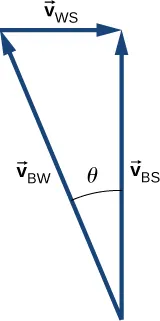d. $|v→BS|=7.4km/h|v→BS|=7.4km/h$ $t=6.5mint=6.5min$
e. $v→BS=8.54km/h,v→BS=8.54km/h,$ but only the component of the velocity straight across the river is used to get the time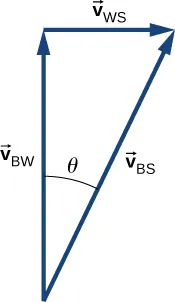$t=6.0mint=6.0min$
Downstream = 0.3 km

77.

$v→AG=v→AC+v→CGv→AG=v→AC+v→CG$
$|v→AC|=25km/h|v→CG|=15km/h|v→AG|=29.15km/h|v→AC|=25km/h|v→CG|=15km/h|v→AG|=29.15km/h$ $v→AG=v→AC+v→CGv→AG=v→AC+v→CG$
The angle between $v→ACv→AC$ and $v→AGv→AG$ is $31°,31°,$ so the direction of the wind is $14°14°$ north of east.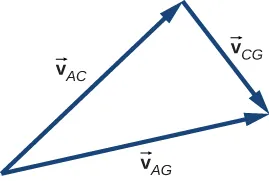79.

$a C = 39.6 m / s 2 a C = 39.6 m / s 2$

81.

$90.0km/h=25.0m/s,9.0km/h=2.5m/s,90.0km/h=25.0m/s,9.0km/h=2.5m/s,$ $60.0km/h=16.7m/s60.0km/h=16.7m/s$
$aT=−2.5m/s2,aC=1.86m/s2,a=3.1m/s2aT=−2.5m/s2,aC=1.86m/s2,a=3.1m/s2$

83.

The radius of the circle of revolution at latitude $λλ$ is $REcosλ.REcosλ.$ The velocity of the body is $2πrT.aC=4π2REcosλT22πrT.aC=4π2REcosλT2$ for $λ=40°,aC=0.26%gλ=40°,aC=0.26%g$

85.

$aT=3.00m/s2aT=3.00m/s2$
$v(5s)=15.00m/saC=150.00m/s2θ=88.8°v(5s)=15.00m/saC=150.00m/s2θ=88.8°$ with respect to the tangent to the circle of revolution directed inward. $|a→|=150.03m/s2|a→|=150.03m/s2$

87.

$a→(t)=−Aω2cosωti^−Aω2sinωtj^a→(t)=−Aω2cosωti^−Aω2sinωtj^$
$aC=5.0mω2ω=0.89rad/saC=5.0mω2ω=0.89rad/s$
$v→(t)=−2.24m/si^−3.87m/sj^v→(t)=−2.24m/si^−3.87m/sj^$

89.

$r → 1 = 1.5 j ^ + 4.0 k ^ r → 2 = Δ r → + r → 1 = 2.5 i ^ + 4.7 j ^ + 2.8 k ^ r → 1 = 1.5 j ^ + 4.0 k ^ r → 2 = Δ r → + r → 1 = 2.5 i ^ + 4.7 j ^ + 2.8 k ^$

91.

$vx(t)=265.0m/svx(t)=265.0m/s$
$vy(t)=20.0m/svy(t)=20.0m/s$
$v→(5.0s)=(265.0i^+20.0j^)m/sv→(5.0s)=(265.0i^+20.0j^)m/s$

93.

$R = 1.07 m R = 1.07 m$

95.

$v 0 = 20.1 m / s v 0 = 20.1 m / s$

97.

$v=3072.5m/sv=3072.5m/s$
$aC=0.223m/s2aC=0.223m/s2$

### Challenge Problems

99.

a. $−400.0m=v0yt−4.9t2359.0m=v0xtt=359.0v0x−400.0=359.0v0yv0x−4.9(359.0v0x)2−400.0m=v0yt−4.9t2359.0m=v0xtt=359.0v0x−400.0=359.0v0yv0x−4.9(359.0v0x)2$
$−400.0=359.0tan40−631,516.9v0x2⇒v0x2=900.6v0x=30.0m/sv0y=v0xtan40=25.2m/s−400.0=359.0tan40−631,516.9v0x2⇒v0x2=900.6v0x=30.0m/sv0y=v0xtan40=25.2m/s$
$v=39.2m/sv=39.2m/s$, b. $t=12.0st=12.0s$

101.

a. $r→TC=(−32+80t)i^+50tj^,|r→TC|2=(−32+80t)2+(50t)2r→TC=(−32+80t)i^+50tj^,|r→TC|2=(−32+80t)2+(50t)2$
$2rdrdt=2(−32+80t)(80)+5000tdrdt=160(−32+80t)+5000t2r=02rdrdt=2(−32+80t)(80)+5000tdrdt=160(−32+80t)+5000t2r=0$
$17800t=5184⇒t=0.29 hr17800t=5184⇒t=0.29 hr$,
b. $|r→TC|=17km|r→TC|=17km$

Order a print copy

As an Amazon Associate we earn from qualifying purchases.